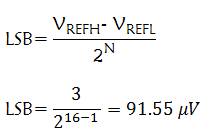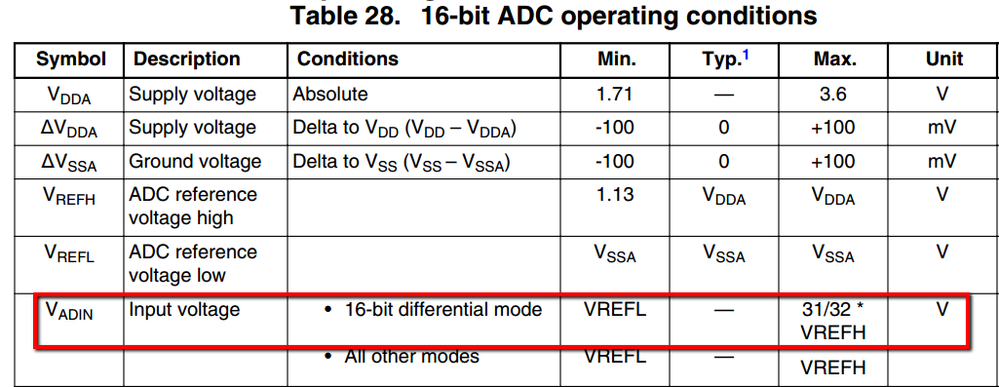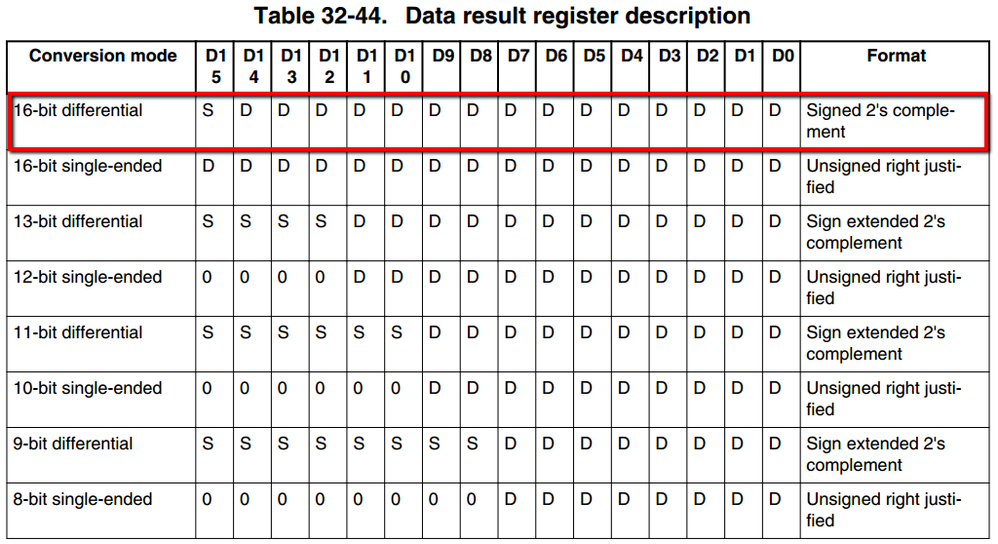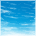## K20 (ADC or DMA issue) ADC results are half of the correct value

cancel
Showing results for
Did you mean:
SOLVED

## K20 (ADC or DMA issue) ADC results are half of the correct value

1,641 ViewsContributor III

I am using the ADC on a K20F120, and getting results that are exactly half what they should be (according to an oscilloscope which I am measuring the input with).  My configuration is as follows:

core clock = 120 MHz
bus clock = 60 MHz

ADC mode = 16 bit differential

ADC trigger = software triggered, free running

ADC triggers DMA transfers on conversion complete

ADC is calibrated before being used

ADC is running at correct speed

No PGA configured

ADC range is 0V-3V (approximately the same as Vdd)

I can post the configuration if that would help (I am not using processor expert), but I am wondering if anyone has encountered this issue before.  It looks as if the results are being right-shifted.  The problem could be something with the ADC or DMA.

Any input would be greatly appreciated. Thanks for reading my post!

Labels (1)
• ### Kinetis K Series MCUs

1 Solution
504 ViewsNXP Employee

Those are actually the expected ADC result. Please be aware that the ADC result is a 16-bit register, therefore the sign will be on D15. For your configuration the ADC result will be around

-32768 when DP = 0 V and DM = 3 V

32767 when DP = 3 V and DM = 0 V

To calculate the LSB you need to use 15-bit resolution because the most significant bit is used like a signUsing 91.55 uV with 1.5V differential input the ADC result will be ~16384

11 Replies
504 ViewsNXP Employee

For the Kinetis 16-bit SAR differential reads means that you have a pair of external pins.

The ADC digital result is the subtraction between the differential inputs

DPx -- Differential input plus

DMx -- Differential input minus

This doesn't means that the ADC accepts negative input voltages, the ADC input voltage range is between VREFL and 31/32 VREFHThe ADC output format is in 2's complement 16-bit signed will be504 ViewsContributor III

Yes, this is exactly what I thought; my VREFL = 0V, and VREFH = 3V DC.  I am wondering if there is anything in my setup causing the results to be divided by two.

My ADC configuration is as follows:

{

}

The DMA is configured as follows:

disable_int(INT);                    // not ready for this interrupt yet. Plug vector first.

DMA_CR = DMA_CR_GRP0PRI_MASK; //writes to the CR register must be performed when the DMA channels are inactive, that is when TCDn_CSR[ACTIVE] bits are cleared

DMA_SOFF_REG(DMA_BASE_PTR,number) = 0;

DMA_ATTR_REG(DMA_BASE_PTR,number) = DMA_ATTR_SMOD(0)             // Source address module disabled

| DMA_ATTR_SSIZE(1)           // 16-bit source data transfer size

| DMA_ATTR_DMOD(0)            // Destination address module disabled

| DMA_ATTR_DSIZE(1);          // 16-bit destination data transfer size

DMA_NBYTES_MLNO_REG(DMA_BASE_PTR,number) = 2;                    // Minor byte transfer count (on minor loop mapping is disabled)

DMA_DOFF_REG(DMA_BASE_PTR,number) = 2;                           // Destination address signed offset

DMA_DLAST_SGA_REG(DMA_BASE_PTR,number) = 0;                       // Adjustment value added to the destination address at the completion of the major iteration count

if(number <16){

}else{

}

enable_int(INT);                     // Enable DMA1 interrupt

504 ViewsSenior Contributor III

I guess we need to be more explicit.  The setting of 'differential mode' means that IN+ can be 3V, IN- 0V, and the reading will (ideally) be 0x7FFF (positive max).  Conversely, IN+ can be 0V, and IN- at 3V (for a 'net' -3V signal) and the 'ideal' reading will be 0x8000 (max negative).  This is all 'not counting' the errata that limits positive voltages to 31/32 of VrefH (2.9V on a 3V reference like REF3030) or full-scale 0x8400 thru 0x7BFF.

504 ViewsContributor III

The maximum reading I am getting is 0x3EA8 (16040) when the input is near Vdd, which is about half of what it should be; the readings are always half of what they should be.  I get similar results for the 'negative' readings.  I am not sure whether this error is being caused by the ADC or DMA configuration.

504 ViewsSenior Contributor III

You say 'the input is near Vdd' but 'differential' requires two inputs, so what is the voltage in this test on the OTHER pin?

504 ViewsContributor III

The other input (my Vref) is at 1.5V, halfway between Vdd and Vss.  It appears Vref is unlikely to be the source of the problem, as I am getting half the readings I should throughout the full range of the ADC.

504 ViewsSenior Contributor III

So if the 'other input' is 1.5V, then the 'differential voltage' measured relative to the 'primary' input is thus at most 1.5V above, or 1.5V below, or half of full scale of +/-3V (a full 16-bit span of 6V), exactly as you report.  To get a 'full scale' differential signal will require one of the inputs at ground, the other at 2.9V.  If you are 'simply biasing' the '-' input at 1.5V, and are NOT 'routing' it along with the '+' input to the ultimate source, in a manner to reject some AC/DC interference, then I strongly suggest you just sample the '+' input in single-ended mode (and invert the MSB to turn it into a sign-bit, so that 'half scale' 0x8000 becomes 0x0000).  I am a little confused by your use of the term Vref -- to the ADC, VrefH is the 'source of scaling reference' against which the analog input voltage (single-ended, or the difference of two inputs in differential mode) is defined.

504 ViewsContributor III

Freescale specifies "VREFH" as the "ADC reference high"; in my case this is 3V, which is also my Vdd.  The "VREFH" is "ADC reference low", and is 0V, and my Vss.  My inputs are both coming from an analog circuit on the PCB (the ultimate source), which has dual-supply amplifiers referred to a 'Vref' level of 1.5V (this is my 'in-') and output a signal called 'signal' which is my in+.  This part of the configuration is working perfectly well.

My issue is that when the voltage difference between 'in-' and 'in+' goes from 0 mV to +1500 mV, the ADC should go from ~0 to ~32000, but the results I am getting are going from ~0 to ~16000.  This is why I say that my problem is that the readings are half what they should be.  There is no problem with voltage offset, or the rest of the circuit, it is simply that the ADC readings are exactly half as large as they should be, through the whole range from 0V-3V (which should be -32000 to 32000 on the ADC, but which I am reading as -16000 to 16000).

504 ViewsSenior Contributor III

The 'whole range', considered differentially, is 6V, from -3V to +3V.

505 ViewsNXP Employee

Those are actually the expected ADC result. Please be aware that the ADC result is a 16-bit register, therefore the sign will be on D15. For your configuration the ADC result will be around

-32768 when DP = 0 V and DM = 3 V

32767 when DP = 3 V and DM = 0 V

To calculate the LSB you need to use 15-bit resolution because the most significant bit is used like a signUsing 91.55 uV with 1.5V differential input the ADC result will be ~16384

504 ViewsSenior Contributor III

"ADC mode = 16 bit differential" means the ADC range is -3V to +3V.